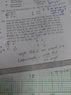# Solve Q14: Linear Dimension Question

• lingkky

#### lingkky

Hello everyone .I cannot understand what Q14 is asking about. May I know how to solve Q14 shown in the pic？

#### Attachments

•IMG-20180520-WA0012.jpg
39.4 KB · Views: 308
The ratio of strength will be the ratio of cross-sectional areas divided by the ratio of mass (the problem says 'weight' but generally in physics it is easier to work with mass). You are told that the ants have the same shape and you can assume they are made of the same density of material. What is the ratio of the area of a triangle to a triangle that is X times bigger by linear dimension, by which we mean that each edge of the second triangle is X times the length of the corresponding edge on the first triangle?

Next imagine two cubes of granite. If the second cube has edges X times the length of those of the first cube, what is the ratio of the mass of the second to that of the first?

@lingkky, you'll have to repost this using the homework template, with an attempt at a solution.